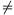Mathematical and Physical Journal
for High Schools
Issued by the MATFUND Foundation
 Already signed up? New to KöMaL?

#Problem C. 826. (November 2005)

C. 826. Solve the following equation:(5 pont)

Deadline expired on December 15, 2005.

Sorry, the solution is available only in Hungarian. Google translation

Alakítsuk az egyenletet (x1):3x3-(x3+x2+x)=2(x3-1),

x2+x-2=0.

x1=-2, x2=1.

A kikötés miatt csak az x=-2 a megoldása az egyenletnek.

### Statistics:

 539 students sent a solution. 5 points: 419 students. 4 points: 57 students. 3 points: 31 students. 2 points: 6 students. 1 point: 2 students. 0 point: 6 students. Unfair, not evaluated: 18 solutionss.

Problems in Mathematics of KöMaL, November 2005